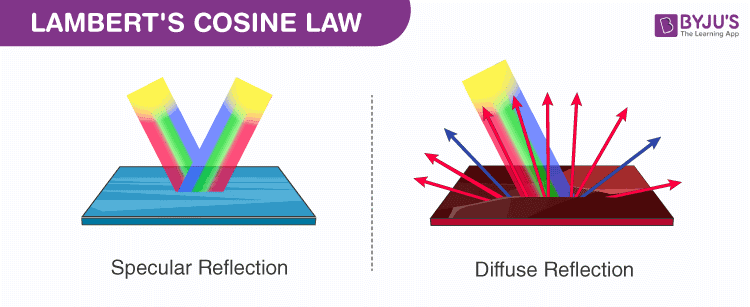# Lambert's Cosine Law

Lambert’s cosine law states that the radiant intensity from the ideal diffusely reflecting surface and cosine of the angle θ between the direction of incident light and surface normal are directly proportional. This law is named after Johann Heinrich Lambert which is studied in optics and also known as Lambert’s emission law or cosine emission law.## Diffuse reflection

Diffuse reflection can be defined as the type of reflection of light or an incident ray where scattering happens at many angles and not just at one angle. Lambertian reflection is the property exhibited by the diffuse reflecting.

Lambertian reflection is defined as the appearance of luminous such that when viewed from all the angles is equal.

This is the main difference between specular reflection and diffuse reflection.

### Examples of diffuse reflection

• Paints: The matte paints that are used in home painting exhibit diffuse reflection while the glossy paints reflect diffusely as well as specular reflection.
• Frosted glass bulb
• Human eye: The primary mechanism of the human eye is to reflect the incident ray in angles which is based on diffuse reflection.

### Luminous flux derivation

Luminous flux is also known as luminous power which is the measure of the power of light perceived.

Luminous flux derivation is as follows

$$F_{tot}=\int_{0}^{\frac{\pi }{2}}\int_{0}^{2\pi }cos(\Theta )I_{max}sin(\Theta )d\phi d\Theta$$ $$=2\pi *I_{max}\int_{0}^{\frac{\pi }{2}}coa(\Theta )sin(\Theta )d\Theta$$ $$=2\pi *I_{max}\int_{0}^{\frac{\pi }{2}}\frac{sin(2\Theta )}{2}d\Theta$$ $$=\pi sr*I_{max}$$

Where,

sinӨ: Jacobian matrix determinant

Imax: luminous flux

To know more on reflection of light, refraction of light and their types, stay tuned with BYJU’S.

Related Physics articles:

Test your knowledge on Lamberts cosine law